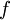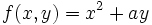# Variable

In mathematics, a variable is a number whose value is not known. In basic arithmetic problems, the variable is typically the answer which the student must find:

Given:
5 + x = 12

Find x.


Since 5 + 7 = 12, the answer is:

x = 7


A variable is usually is the subject or part of a function and must be subject to change while not affecting the structure behind the function in which it acts.

For example, if we have a function:, thenare said to be the variables of the function,.

Further,. We change the variable and thus alter the value of the function.

Sometimes a function can have also a parameter. A parameter is similar to a variable, but is not written like a variable and is thus more similar to a constant. For example, if we a function:, thenis said to be a parameter. It does not change like the variables x and y, but it can nonetheless take different values. It is important to clearly distinguish the two concepts.

The set of all variables upon which a function acts is said to be the domain. The set of all values that a function can take due to its domain is called its range.